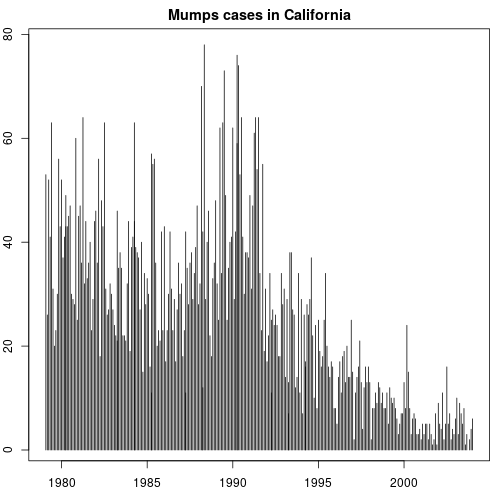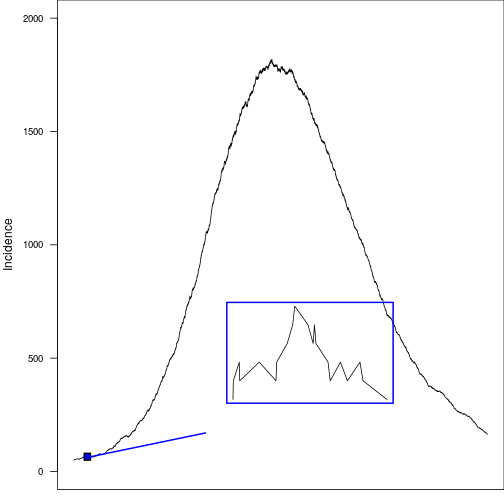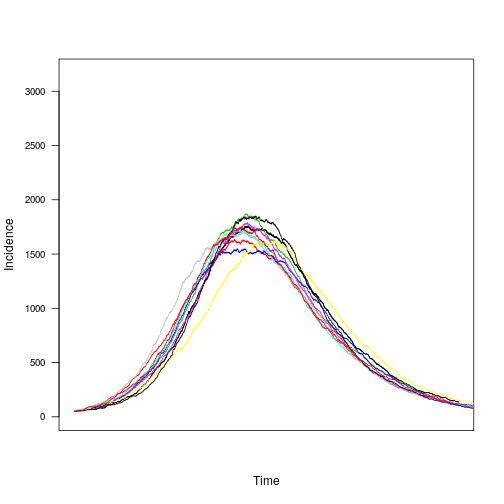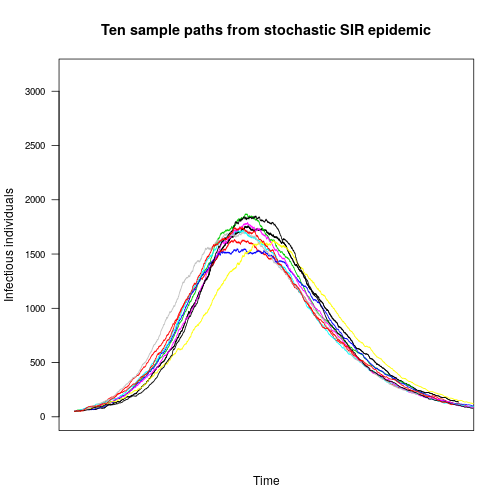# Stochastic models

John M. Drake & Pej Rohani

### Epidemiological data are variable/noisy### Epidemiological data are variable/noisy

Noise is addressed using stochastic models

Two types of noise:

• Observation error: the data are probabilistically related to the true state of the system
• Process noise: the system progresses probabilistically

We distinguish further between two types of process noise:

• Environmental stochasticity: some parameter is a random variable
• Demographic stochasticity: individual-level chance events

Discussion question. What are the relationships among the following words/concepts: error, noise, probabilistic, random, stochastic, variability/variation?

### The SIR model is a continuum approximationThe $$SIR$$ model, $$dY/dt = \beta XY/N - \gamma Y$$, implies that changes in the states $$X$$, $$Y$$, and $$Z$$ are continuous. But, in reality individuals are either susceptible, infected, or recovered so that $$X$$, $$Y$$, and $$Z$$ are integer-valued and changes in the system state occur as discrete steps. The differential equation is an idealization.

### Demographic stochasticity

• What we seek is a stochastic model for which the system of ODEs is an appropriate idealization
• There are an infinite number of such models, but the simplest one is a continuous-time, discrete-space Markov Chain with propensities given by the various terms in the differential equations
• This model may also be interpreted as an event-driven model with state transition probabilities

Propensities

Rate Transition
$$\beta XY/N$$ $$X \rightarrow X-1$$, $$Y \rightarrow Y+1$$
$$\gamma Y$$ $$Y \rightarrow Y-1$$, $$Z \rightarrow Z+1$$

### Other kinds of stochastic models

1. Continuous and discrete time branching processes
2. Stochastic differential equations may be found as a continuum approximation to a discrete space model or to model environmental stochasticity
3. Reed-Frost model
4. Agent-based models, etc.

A stochastic differential equation (SDE):

$dX(t) = f(t) X(t) dt + h(t) X(t) dW(t)$

SDEs require stochastic calculus (Ito or Stratonovich) to solve.

Note: Lots of scientific papers gloss over the mathematical details of the models they report, but best practice is to be explicit.

### Writing down the model mathematically

The Master Equation is a system of differential equations for the flow in the probability that he system will be in any given (multi-dimensional) state

$dP_k/dt = \sum_l A_{kl}P_l$

where $$A$$ is a matrix of transition propensities.

This approach is only tractable for very simple models (e.g. $$SI$$ and $$SIS$$ epidemics)

### Simulating the model

Exact simulation is straightforward via Gillespie's Direct method. To simulate a sample path:

1. Initialize
2. Iteration of a two step process
• Determine time of the next event
• Determine change of state at the next event time
3. Summarize

### Step 1. Time to next event

Given system state $$N$$, let $$R(N)$$ be the sum of all the propensities for all changes of state and $$G_N(s)$$ be the probability that no event occurs in subsequent time interval $$s$$ for system state $$N$$.

By the Markov assumption

\tiny{ \begin{aligned} G_N(s + \delta s) &= Pr \left\{ \mbox{no event in } (t, t+s+\delta s) \right\} \\ &= Pr \left\{ \mbox{no event in } (t, t+s) \right\} \times Pr \left\{ \mbox{no event in } (t+s, t+s+\delta s) \right\} \\ &= G_N(s) \times \left\{ 1-R(N) \times \delta s \right\} \end{aligned} }

### Step 1. Time to next event

After rearranging

\begin{align*} \frac{G_N(s + \delta s) - G_N(s)}{\delta s} &= -R(N) \times G_N(s) \end{align*}

Letting $$\delta s \to 0$$

\begin{align*} \frac{d G_N}{d s} &= -R(N) \times G_N(s) \end{align*}

With solution

\begin{align*} G_N(s) &= e^{-R(N)s} \end{align*}

Thus, the probability the next event occurs in $$(t, t+s)$$ is

\begin{align*} F_N(s) &= 1- e^{-R(N)s} \end{align*}

### Step 1. Time to next event

Given event time distribution $$F_N$$, an exponentially distributed random event time $$S$$ can be obtained from a uniform random random variate $$U_1$$ by setting

\begin{align*} U_1 = F_N(s) = 1- e^{-R(N)S} \end{align*}

and solving to obtain

\begin{align*} S = - \log(U_1) /R(N) \end{align*}

### Step 2: change of state

Let the propensities of event types $$E_1, E_2, E_3, ...$$ be denoted $$R_1, R_2, R_3, ....$$ with total rate $$R_{sum} = R(N)=\sum_i R_i$$. In the long run, events of each type should occur with relative frequency $$R_i/R(N)$$. We can randomly draw event classes with these frequencies by simulating a second uniform random variate $$U_2$$ and assigning event class $$E_i$$ if

\begin{align*} R_{sum}^{-1}\sum_{i=1}^{p-1} R_i < U_2 \leq R_{sum}^{-1}\sum_{i=1}^{p} R_i. \end{align*}

### Gillespie's direct method

1. Label all possible events $$E_1, E_2, E_3, ...$$
2. Initialize $$t=0$$ and state $$N$$
3. Update step
1. Calculate propensities $$R_1, R_2, R_3, ...$$
2. Calculate $$R_{sum} = R(N)=\sum_i R_i$$
3. Generate $$U_1$$ and transform to obtain $$S$$
4. Generate $$U_2$$ and determine event type $$E_i$$
5. Update state based on $$E_i$$
6. Update time $$t=t+S$$
4. Go to step (3)

### Example with SIR model

Events

• $$E_1$$: Birth of susceptible individual ($$X \to X+1$$)
• $$E_2$$: Infection ($$X \to X-1, Y \to Y+1$$)
• $$E_3$$: Recovery ($$Y \to Y-1, Z \to Z+1$$)
• $$E_4$$: Death of susceptible individual ($$X \to X-1$$)
• $$E_5$$: Death of infected individual ($$Y \to Y-1$$)
• $$E_6$$: Death of recovered individual ($$Z \to Z-1$$)

### Example with SIR model

Propensities

• $$R_1$$: $$\mu (X+Y+Z)$$
• $$R_2$$: $$\beta XY/N$$
• $$R_3$$: $$\gamma Y$$
• $$R_4$$: $$\mu X$$
• $$R_5$$: $$\mu y$$
• $$R_6$$: $$\mu Z$$

### Example R code

Function SIR.onestep performs calculations of each update step

SIR.onestep <- function (x, params) {
X <- x; Y <- x; Z <- x ;N <- X+Y+Z
with(
as.list(params),
{
rates <- c(mu*N, beta*X*Y/N, mu*X, mu*Y, gamma*Y, mu*Z)
changes <- matrix(c( 1, 0, 0,
-1, 1, 0,
-1, 0, 0,
0,-1, 0,
0,-1, 1,
0, 0,-1),
ncol=3, byrow=TRUE)
U1<-runif(1); tau<--log(U1)/sum(rates)
U2<-runif(1)
m<-min(which(cumsum(rates)>=U2*sum(rates)))
x<-x[2:4] + changes[m,]
return(out <- c(tau, x))
}
)
}


### Example R code

Now we write a function SIR.model that iteratively calls SIR.onestep to simulate an epidemic

SIR.model <- function (x, params, nstep) {  #function to simulate stochastic SIR
output <- array(dim=c(nstep+1,4))         #set up array to store results
colnames(output) <- c("time","X","Y","Z") #name variables
output[1,] <- x                           #first record of output is initial condition
for (k in 1:nstep) {                      #iterate for nstep steps
output[k+1,] <- x <- SIR.onestep(x,params)
}
output                                    #return output
}


### Example R code

Finally, we write a code that calls SIR.model to simulate epidemics

set.seed(38499583)                #set seed
nsims <- 10                       #number of simulations
pop.size <- 10000                   #total population size
Y0 <- 50                           #initial number infected
X0 <- round(0.98*pop.size)        #initial number suscepitlble (~98% of population)
nstep <- 16000                     #number of events to simulate
xstart <- c(time=0,X=X0,Y=Y0,Z=pop.size-X0-Y0) #initial conditions
params <- list(mu=0.00001,beta=60,gamma=365/13) #parameters
data <- vector(mode='list',length=nsims) #initialize list to store the output
for (k in 1:nsims) {              #simulate nsims times
data[[k]] <- as.data.frame(SIR.model(xstart,params,nstep))
data[[k]]$cum.time <- cumsum(data[[k]]$time)
}
max.time<-data[]$cum.time[max(which(data[]$Y>0))] #maximum time in first simulation
max.y<-1.8*max(data[]\$Y)       #find max infected in run 1 and increase by 80% for plot
plot(Y~cum.time,data=data[],xlab='Time',ylab='Incidence',col=1,xlim=c(0,max.time),ylim=c(0,max.y), type='l', axes=FALSE)
box()
axis(2, cex.axis=0.8, las=2)

for (k in 1:nsims) {              #add multiple epidemics to plot
lines(Y~cum.time,data=data[[k]],col=k,type='l')
}### Example R code### Stochastic phenomena

• Extinction
• The J to U transition